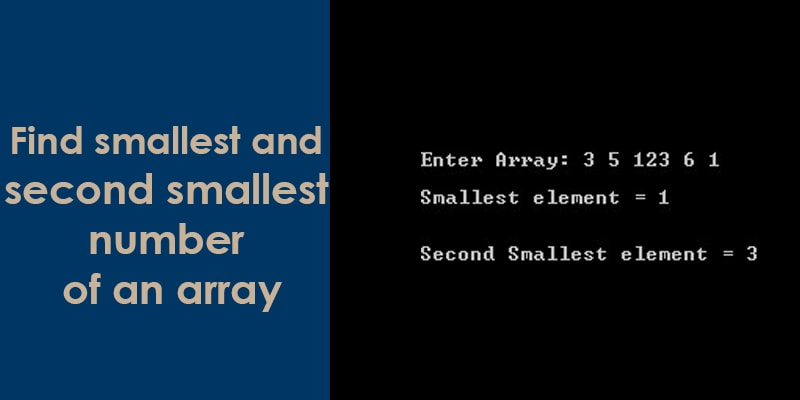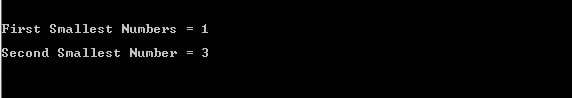# Find the smallest and second smallest element in an array using c languageWe can find the smallest and second smallest number of an integers array using the c language. This question is also important and asks by the interviewer in an interview.

Let’s take an example, suppose here is an integer array iaData of 5 integers.

int iaData = {3, 5, 123, 6, 1};
Here the smallest number is 1 and the second smallest number is 3.

A simple way to find the smallest and second smallest number is that sort the array in ascending order and pick its first and second elements. Its first element will be the smallest number and the second number will be the second smallest number. The time complexity of this solution is O(n Log n).

OutPut:Above method is not better because it scans the array twice. There is also another way to find the smallest and second smallest number in a single scan of the array. The time complexity of this solution is O(n).

Here is an algorithm to find two smallest and second smallest number in one traversal.

#### The algorithm to find smallest and second smallest number:

• Create two variable iFirstSmallest and iSecondSmallest.
• Initialize both variables as INT_MAX
iFirstSmallest = INT_MAX
iSecondSmallest = INT_MAX
• Loop through all the elements and perform the below task
1). If the current element is smaller than iFirstSmallest, then update iFirstSmallest and iSecondSmallest.
2). Else if the current element is smaller than iSecondSmallest then update iSecondSmallest.

OutPut: